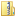# Amplifying the Block Matrix Structure for Spectral Clustering

This page contains reference data and code used in a paper by Jan Poland and myself, published back in 2005.

## Data Sets Used

This benchmark data for spectral clustering algorithms. are organized in Matlab .mat files, each containing the following variables:

VariableMeaning
x the matrix of the data themselves, as column vectors
c0 a row vector of correct cluster assignments, an int for each point
nn a row vector of cluster sizes, an int for each cluster
sgm a matrix of best kernel widths (sigma) for every algorithm. Each row contains five empirically found best values for the corresponding algorithm. The algorithms are ordered as follows:
1. KEM
2. Ng & al.
3. Basic spectral
4. Conductivity
5. Laplacian
sgmHist a vector of most probable sigmas according to the histogram method

The data sets are:

## Programs Used

The algorithms were implemented---with one exception for performance reasons---in Matlab. The programs files are:

You may download all the files as a single zip file.